# Need help with vectors question

[Solved! Thanks Tim!] Need help with vectors question

## Homework Statement

The line L has cartesian equations x-2 = (y-5)/2 = -z. Find the perpendicular distance from the origin to L.

Find also the acute angle between L and the z-axis.

## The Attempt at a Solution

Sorry, pretty much in a rush to finish this soon as I have been stuck at understanding this vectors chapter for a really long time. I hope someone will be able to help with it...I've tried but I really cannot figure it out alone.

I don't know what concepts I am missing out on but I am stuck.

Help will be much appreciated. I'm trying to learn and not just get answers. Rushing on revision as I don't have much time...planning to finish my math revision in 2 months.

All I know is the fact that if vectors a and b are perpendicular to each other their product of directional vectors will be zero, but that's all. And it is not helpful.

Last edited:

tiny-tim
Homework Helper
All I know is the fact that if vectors a and b are perpendicular to each other their product of directional vectors will be zero, but that's all. And it is not helpful.

Hi PuzzledMe!Hint: What is the equation of the line through the origin parallel to L?

What lines are perpendicular to this new line?

So what is the perpendicular plane?

Where does that plane intersect L?Sorry, pretty much in a rush to finish this soon as I have been stuck at understanding this vectors chapter for a really long time. I hope someone will be able to help with it...I've tried but I really cannot figure it out alone.
I don't know what concepts I am missing out on but I am stuck.
Help will be much appreciated. I'm trying to learn and not just get answers. Rushing on revision as I don't have much time...planning to finish my math revision in 2 months.
All I know is the fact that if vectors a and b are perpendicular to each other their product of directional vectors will be zero, but that's all. And it is not helpful.
If you spent less type talking and more with your work, you would get more help. Show your attempts!!!

Ok I just got the pics of my working: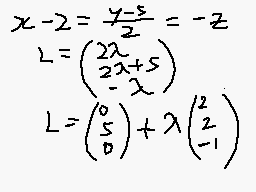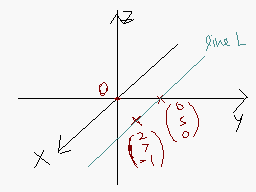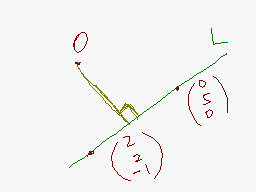I'm still solving it and reflecting on tips given by tim (thanks tim, will get back once I've worked it out on my own). But I do appreciate it if someone can point out if I'm on the right track.

What is the equation of the line through the origin parallel to L?
Equation of line passing through origin parallel to L = (0i + 0j + 0k) + λ(2i + 2j -1k)

What lines are perpendicular to this new line?
So what is the perpendicular plane?
Where does that plane intersect L?
--- > Thinking..

Last edited:
tiny-tim
Homework Helper
Hi PuzzledMe!warning! warning! emergency!Yes, your way isn't quite the same as mine, but it's just as good!But (0,5,0) isn't on L!(Just put in the numbers!)

Hi PuzzledMe!warning! warning! emergency!Yes, your way isn't quite the same as mine, but it's just as good!But (0,5,0) isn't on L!(Just put in the numbers!)
Ah shucks so something was wrong with what I'm thinking about 3d vectors...
So it should look something like this?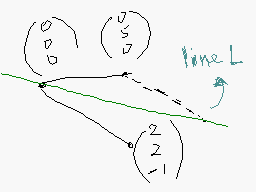Equation of line passing through origin parallel to L = (2i + 7j -1k) --- > Is this right?

But then I don't know where the perpendicular will be...

tiny-tim
Homework Helper
… don't panic … !

Whoa! You're panicking!

The method was right. You found a point on the line, and added lambda times any number for any other point.

Any point on the line will do … it doesn't need to be a special point, like on one of the axes … you don't need a diagram.

Just put z = 0 (or x = 19, or x + y = -1107, or …, but z = 0 is easier!). Then x = … and y = … , which gives you (?,?,0), and you just carry on as before.My bad, I see the problem, careless mistake.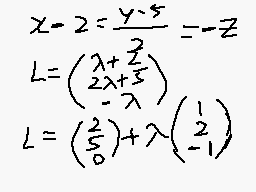Still thinking about how to go about finding the perpendicular line equation here...

tiny-tim
Homework Helper
But you're there!

If the foot of the perpendicular is P = (2,5,0) + µ(1,2,-1),
then what do you know about the line OP?P must be a point on L.

But then that line OP has to be perpendicular to L. Which is the part I'm stuck as I can't find the appropriate equation to describe it...

Last edited:
tiny-tim
Homework HelperOP.L = 0OP = (a,b,c)
OP.L = 0
(a,b,c).(1,2,-1)=0

a + 2b - c = 0

Here are my other stupid workings here and I don't know how much it relates to the question but I'll definitely try anything that may work...

|(1,2,-2)| = √9 = 3
cos θ = f.g / [|f||g|]
cos 90˚ = 0 / [3 x (√a² + b² + c²)]
0 = 0 / [3 x (√a² + b² + c²)]
[3 x (√a² + b² + c²)] = 0
(√a² + b² + c²) = 0

tiny-tim
Homework Helper
PuzzledMe, you're puzzling me!

It's no good saying (a,b,c) = P and finding one equation for a b and c.

You need to know what all of them are.

Which you do by using your original formula for P.

P = (2,5,0) + µ(1,2,-1).

So OP = (2,5,0) + µ(1,2,-1).

And L is parallel to OL´, where L´ = (1,2,-1).

So 0 = OP.OL´ = … ?

So µ = … ?I'm almost puzzled by certain kinds of maths, that's what my name stands for and how I chose it T.T
Tend to think too far off, sorry.

Ok I follow you, I got it for the first part, thanks for being patient with me!

0 = OP.L´
= (2+µ, 5+2µ, -u).(1, 2, -1)

2 + µ + 10 + 4µ + µ = 0
12 + 6µ = 0
6µ = -12
µ = -2

OP = (2, 5, 0) - 2(1, 2, -1)
= (0, 1, 2)

Length of OP = (√1² + 2²) = √5

Thanks again! I'll try the next one on my own first I guess =X

Problem solved!

L = (2, 5, 0) + λ(1, 2, -1).
Equation of z-axis = (0, 0, 0) + α (0, 0, 1)

Let acute angle be â.

cos â = [(1, 2, -1).(0, 0, 1)] / {[√1² + 2² + (-1)²] x (√1)}
cos â = 1 / (√6)
â = 65.9°

Thanks =)